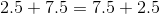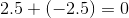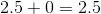# SSAT Middle Level Math : How to add fractions

## Example Questions

### Example Question #336 : Fractions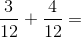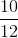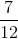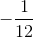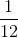Explanation:

Add the numerators and leave the denominators the same: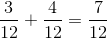Answer:### Example Question #337 : Fractions

Solve: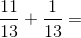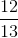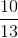Explanation:

Add the numerators and leave the denominators the same: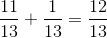Answer:### Example Question #338 : Fractions

Solve: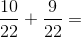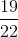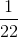Explanation:

Add the numerators and leave the denominators the same: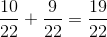Answer:### Example Question #339 : Fractions

Solve: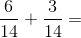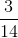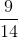Explanation:

Add the numerators and leave the denominators the same: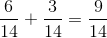Answer:### Example Question #340 : Fractions

Which of the following is the sum of sixty-seven tenths, sixty-seven hundredths, and sixty-seven ten-thousandths?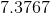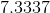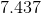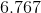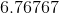Explanation:

Sixty-seven tenths, sixty-seven hundredths, and sixty-seven ten-thousandths can be rewritten as 6.7, 0.67, and 0.0067, respectively. Add, appending zeroes at the end of each number as needed: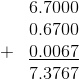### Example Question #41 : How To Add Fractions

Which of the following is the sum of two fifths, one fourth, and three tenths?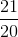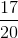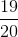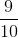Explanation:

We must express the three fractions as their equivalents with a common denominator. Since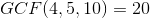, we rewrite each fraction in terms of twentieths, then add as follows: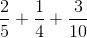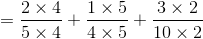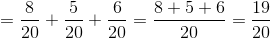### Example Question #441 : Concepts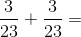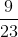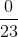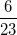Explanation:

Add the numerators and leave the denominators alone: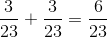Answer:### Example Question #442 : Concepts

Subtract: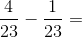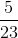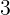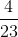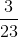Explanation:

Subtract the numerators and leave the denominators alone: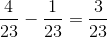Answer:### Example Question #443 : Concepts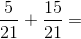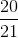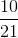Explanation:

Because the two fractions have the same denominator, simply add the numerators and leave the denominator as is: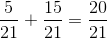Answer:### Example Question #51 : How To Add Fractions

Which of the following statements demonstrates the commutative property of addition?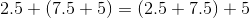None of the examples in the other responses demonstrates the commutative property of addition.